# Tag: permutation representation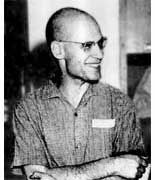The Oscar in
the category The Best Rejected Research Proposal in Mathematics
(ever)
goes to … Alexander Grothendieck
for his proposal Esquisse d’un Programme, Grothendieck\’s research program from 1983, written as
part of his application for a position at the CNRS, the French
equivalent of the NSF. An English translation is
available.

Here is one of the problems discussed :
Give TWO non-trivial elements of
$Gal(\overline{\mathbb{Q}}/\mathbb{Q})$
the _absolute_
Galois group of the algebraic closure of the rational numbers
$\overline{\mathbb{Q}}$, that is the group of all
$\mathbb{Q}$-automorphisms of $\overline{\mathbb{Q}}$. One element
most of us can give (complex-conjugation) but to find any other
element turns out to be an extremely difficult task.

To get a handle on
this problem, Grothendieck introduced his _’Dessins d’enfants’_
(Children’s drawings). Recall from last session the pictures of the
left and right handed Monsieur Mathieu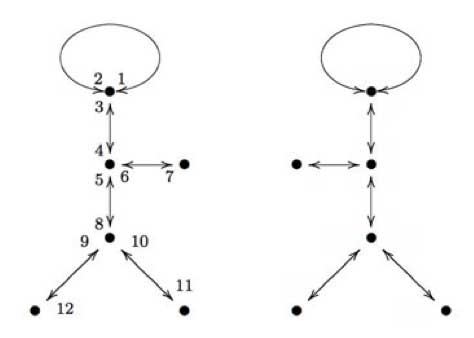The left hand side drawing was associated to a map
$\mathbb{P}^1_{\mathbb{C}} \rightarrow \mathbb{P}^1_{\mathbb{C}}$ which was
defined over the field $\mathbb{Q} \sqrt{-11}$ whereas the right side
drawing was associated to the map given when one applies to all
coefficients the unique non-trivial automorphism in the Galois group
$Gal(\mathbb{Q}\sqrt{-11}/\mathbb{Q})$ (which is
complex-conjugation). Hence, the Galois group
$Gal(\mathbb{Q}\sqrt{-11}/\mathbb{Q})$ acts _faithfully_ on the
drawings associated to maps $\mathbb{P}^1_{\mathbb{Q}\sqrt{-11}} \rightarrow \mathbb{P}^1_{\mathbb{Q}\sqrt{-11}}$ which are ramified only over
the points ${ 0,1,\infty }$.

Grothendieck’s idea was to
extend this to more general maps. Assume that a projective smooth curve
(a Riemann surface) X is defined over the algebraic numbers
$\overline{\mathbb{Q}}$ and assume that there is a map $X \rightarrow \mathbb{P}^1_{\mathbb{C}}$ ramified only over the points
${ 0,1,\infty }$, then we can repeat the procedure of last time and
draw a picture on X consisting of d edges (where d is the degree
of the map, that is the number of points lying over another point of
$\mathbb{P}^1_{\mathbb{C}}$) between white resp. black points (the
points of X lying over 1 (resp. over 0)).

Call such a drawing a
‘dessin d\’enfant’ and look at the collection of ALL dessins
d’enfants associated to ALL such maps where X runs over ALL curves
defined over $\overline{\mathbb{Q}}$. On this set, there is an action
of the absolute Galois group
$Gal(\overline{\mathbb{Q}}/\mathbb{Q})$ and if this action would be
faithful, then this would give us insight into this
group. However, at that time even the existence of a map $X \rightarrow \mathbb{P}^1$ ramified in the three points ${ 0,1,\infty }$
seemed troublesome to prove, as Grothendieck recalls in his proposal

In more erudite terms, could it be true that
every projective non-singular algebraic curve defined over a number
field occurs as a possible ‚ modular curve‚ parametrising
elliptic curves equipped with a suitable rigidification? Such a
supposition seemed so crazy that I was almost embarrassed to submit
it to the competent people in the domain. Deligne when I consulted
him found it crazy indeed, but didn’t have any counterexample up
his sleeve. Less than a year later, at the International Congress in
Helsinki, the Soviet mathematician Bielyi announced exactly that result,
with a proof of disconcerting simplicity which fit into two little
pages of a letter of Deligne ‚ never, without a doubt, was such a
deep and disconcerting result proved in so few lines!

In
the form in which Bielyi states it, his result essentially says that
every algebraic curve defined over a number field can be obtained as
a covering of the projective line ramified only over the points 0,
1 and infinity. This result seems to have remained more or less
unobserved. Yet, it appears to me to have considerable importance. To
me, its essential message is that there is a profound identity
between the combinatorics of finite maps on the one hand, and the
geometry of algebraic curves defined over number fields on the
other. This deep result, together with the algebraic- geometric
interpretation of maps, opens the door onto a new, unexplored world within reach of all, who pass by without seeing it.

Belyi’s proof is indeed relatively easy
(full details can be found in the paper Dessins d’enfants on the
Riemann sphere
by Leila
Schneps). Roughly it goes as follows : as both X and the map are
defined over $\overline{\mathbb{Q}}$ the map is only ramified over
(finitely many) $\overline{\mathbb{Q}}$-points. Let S be the finite
set of all Galois-conjugates of these points and consider the polynomial

$f_0(z_0) = \prod_{s \in S} (z_0 -s) \in \mathbb{Q}[z_0]$

Now, do a
resultant trick. Consider the
polynomial $f_1(z_1) = Res_{z_0}(\frac{d f_0}{d z_0},f_0(z_0)-z_1)$ then the roots of $f_1(z_1)$ are exactly the
finite critical values of $f_0$, $f_1$ is again defined over
$\mathbb{Q}$ and has lower degree (in $z_1$) than $f_0$ in $z_1$.
Continue this trick a finite number of times untill you have constructed
a polynomial $f_n(z_n) \in \mathbb{Q}[z_n]$ of degree zero.

Composing
the original map with the maps $f_j$ in succession yields that all
ramified points of this composition are
$\mathbb{Q}$-points! Now, we only have to limit the number of
these ramified $\mathbb{Q}$-points (let us call this set T) to three.

Take any three elements of T, then there always exist integers $m,n \in \mathbb{Z}$ such that the three points go under a linear
fractional transformation (a Moebius-function associated to a matrix in
$PGL_2(\mathbb{Q})$) to ${ 0,\frac{m}{m+n},1 }$. Under the
transformation $z \rightarrow \frac{(m+n)^{m+n}}{m^m n^n}z^m(1-z)^n$ the points 0 and 1 go to 0 and
$\frac{m}{m+n}$ goes to 1 whence the ramified points of the
composition are one less in number than T. Continuing in this way we
can get the set of ramified $\mathbb{Q}$-points of a composition at
most having three elements and then a final Moebius transformation gets
them to ${ 0,1,\infty }$, done!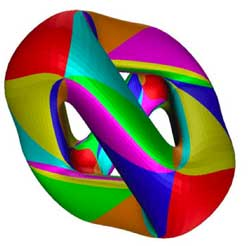As a tribute for this clever
argument, maps $X \rightarrow \mathbb{P}^1$ ramified only in 0,1 and
$\infty$ are now called Belyi morphisms. Here is an example of
a Belyi-morphism (and the corresponding dessin d’enfants) associated to
one of the most famous higher genus curves around : the Klein
quartic
(if you haven’t done
so yet, take your time to go through this marvelous pre-blog post by
John Baez).

One can define the Klein quartic as the plane projective
curve K with defining equation in
$\mathbb{P}^2_{\\mathbb{C}}$ given by $X^3Y+Y^3Z+Z^3X = 0$ K has
a large group of automorphism, namely the simple group of order
168 $G = PSL_2(\mathbb{F}_7) = SL_3(\mathbb{F}_2)$ It is a classical fact (see for example
the excellent paper by Noam Elkies The Klein quartic in number theory) that the quotient map $K \rightarrow K/G = \mathbb{P}^1_{\mathbb{C}}$ is ramified only in the points
0,1728 and $\infty$ and the number of points of K lying over them
are resp. 56, 84 and 24. Now, compose this map with the Moebius
transormation taking ${ 0,1728,\infty } \rightarrow { 0,1,\infty }$
then the resulting map is a Belyi-map for the Klein quartic. A
topological construction of the Klein quartic is fitting 24 heptagons
together so that three meet in each vertex, see below for the gluing
data-picture in the hyperbolic plane : the different heptagons are given
a number but they appear several times telling how they must fit
together)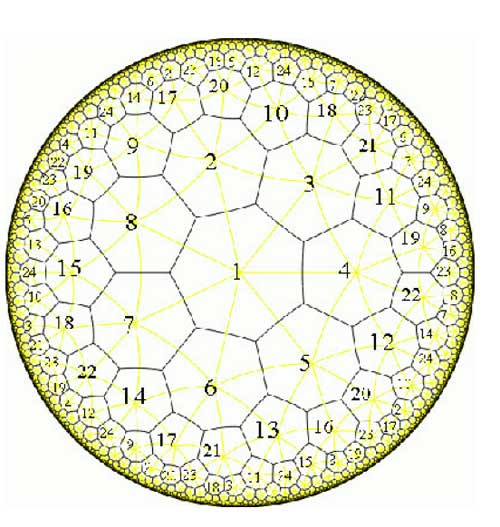The resulting figure has exactly $\frac{7 \times 24}{2} = 84$ edges and the 84 points of K lying over 1 (the white points in
the dessin) correspond to the midpoints of the edges. There are exactly
$\frac{7 \times 24}{3}=56$ vertices corresponding to the 56 points
lying over 0 (the black points in the dessin). Hence, the dessin
d\’enfant associated to the Klein quartic is the figure traced out by
the edges on K. Giving each of the 168 half-edges a
different number one assigns to the white points a permutation of order
two and to the three-valent black-points a permutation of order three,
whence to the Belyi map of the Klein quartic corresponds a
168-dimensional permutation representation of $SL_2(\mathbb{Z})$,
which is not so surprising as the group of automorphisms is
$PSL_2(\mathbb{F}_7)$ and the permutation representation is just the
regular representation of this group.

Next time we will see how
one can always associate to a curve defined over
$\overline{\mathbb{Q}}$ a permutation representation (via the Belyi
map and its dessin) of one of the congruence subgroups $\Gamma(2)$ or
$\Gamma_0(2)$ or of $SL_2(\mathbb{Z})$ itself.

Even a virtual course needs an opening line, so here it is : Take your favourite $SL_2(\mathbb{Z})$-representation Here is mine : the permutation presentation of the Mathieu group(s). Emile Leonard Mathieu is remembered especially for his discovery (in 1861 and 1873) of five sporadic simple groups named after him, the Mathieu groups $M_{11},M_{12},M_{22},M_{23}$ and $M_{24}$. These were studied in his thesis on transitive functions. He had a refreshingly direct style
of writing. I’m not sure what Cauchy would have thought (Cauchy died in 1857) about this ‘acknowledgement’ in his 1861-paper in which Mathieu describes $M_{12}$ and claims the construction of $M_{24}$.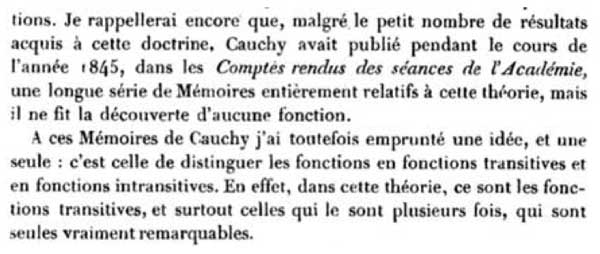Also the opening sentenses of his 1873 paper are nice, something along the lines of “if no expert was able to fill in the details of my claims made twelve years ago, I’d better do it myself”.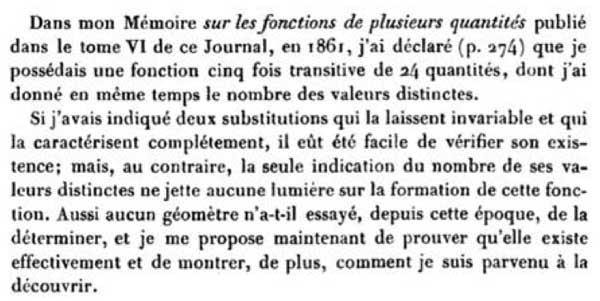However, even after this paper opinions remained divided on the issue whether or not he did really achieve his goal, and the matter was settled decisively by Ernst Witt connecting the Mathieu groups to Steiner systems (if I recall well from Mark Ronan’s book Symmetry and the monster)

As Mathieu observed, the quickest way to describe these groups would be to give generators, but as these groups are generated by two permutations on 12 respectively 24 elements, we need to have a mnemotechnic approach to be able to reconstruct them whenever needed.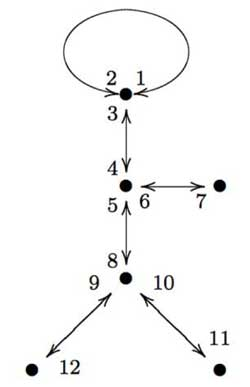Here is a nice approach, due to Gunther Malle in a Luminy talk in 1993 on “Dessins d’enfants” (more about them later). Consider the drawing of “Monsieur Mathieu” on the left. That is, draw the left-handed bandit picture on 6 edges and vertices, divide each edge into two and give numbers to both parts (the actual numbering is up to you, but for definiteness let us choose the one on the left). Then, $M_{12}$ is generated by the order two permutation describing the labeling of both parts of the edges

$s=(1,2)(3,4)(5,8)(7,6)(9,12)(11,10)$

together with the order three permutation obtained from cycling counterclockwise
around a trivalent vertex and calling out the labels one encounters. For example, the three cycle corresponding to the ‘neck vertex’ is $~(1,2,3)$ and the total permutation
is

$t=(1,2,3)(4,5,6)(8,9,10)$

A quick verification using GAP tells that these elements do indeed generate a simple group of order 95040.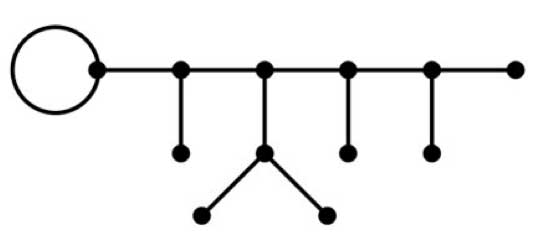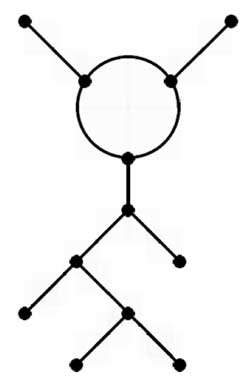Similarly, if you have to reconstruct the largest Mathieu group from scratch, apply the same method to the the picture above or to “ET Mathieu” drawing on the left. This picture I copied from Alexander Zvonkin‘s paper How to draw a group as well as the computational details below.

This is all very nice and well but what do these drawings have to do with Grothendieck’s “dessins d’enfants”? Consider the map from the projective line onto itself

$\mathbb{P}^1_{\mathbb{C}} \rightarrow \mathbb{P}^1_{\mathbb{C}}$

defined by the rational map

$f(z) = \frac{(z^3-z^2+az+b)^3(z^3+cz^2+dz+e)}{Kz}$

where N. Magot calculated that

$a=\frac{107+7 \sqrt{-11}}{486}, b=-\frac{13}{567}a+\frac{5}{1701}, c=-\frac{17}{9}, d=\frac{23}{7}a+\frac{256}{567}, e=-\frac{1573}{567}a+\frac{605}{1701}$

and finally

$K = -\frac{16192}{301327047}a+\frac{10880}{903981141}$

One verifies that this map is 12 to 1 everywhere except over the points ${ 0,1,\infty }$ (that is, there are precisely 12 points mapping under f to a given point of $\mathbb{P}^1_{\mathbb{C}} – { 0,1,\infty }$. From the expression of f(z) it is clear that over 0 there lie 6 points (3 of which with multiplicity three, the others of multiplicity one). Over $\infty$ there are two points, one with multiplicity 11 and one with multiplicity one. The difficult part is to compute the points lying over 1. The miraculous fact of the given values is that

$f(z)-1 = \frac{-B(z)^2}{Kz}$

where

$B(z)=z^6+\frac{1}{11}(10c-8)z^5+(5a+9d-7c)z^4+(2b+4ac+8e-6d)z^3+$
$(3ad+bc-5e)z^2+2aez-be)$

and hence there are 6 points lying over 1 each with mutiplicity two.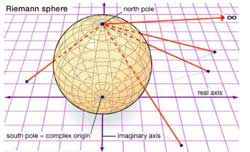Right, now consider the complex projective line $\mathbb{P}^1_{\mathbb{C}}$ as the Riemann sphere $S^2$ and mark the six points lying over 1 by a white vertex and the six points lying over 0 with a black vertex (in the source sphere). Now, lift the real interval $[0,1]$ in the target sphere $\mathbb{P}^1_{\mathbb{C}} = S^2$ to its inverse image on the source sphere. As there are exactly 12 points lying over each real
number $0 \lneq r \lneq 1$, this inverse image will consist of 12 edges which are noncrossing and each end in one black and one white vertex. The obtained graph will look like the \”Monsieur Mathieu\” drawing above with the vertices corresponding to the black vertices and the three points over 1 of multiplicity three corresponding to the
trivalent vertices, those of multiplicity one to the three end-vertices. The white vertices correspond to mid-points of the six edges, so that we do get a drawing with twelve edges, one corresponding to each number. From the explicit description of f(z) it is clear that this map is defined over $\mathbb{Q}\sqrt{-11}$ which is also the
smallest field containing all character-values of the Mathieu group $M_{12}$. Further, the Galois group of the extension $Gal(\mathbb{Q}\sqrt{-11}/\mathbb{Q}) = \mathbb{Z}/2\mathbb{Z}$ and is generated by complex conjugation. So, one might wonder what would happen if we replaced in the definition of the rational map f(z) the value of a by $a = \frac{107-\sqrt{-11}}{486}$. It turns out that this modified map has the same properties as $f(z)$ so again one can draw on the source sphere a picture consisting of twelve edges each ending in a white and black vertex.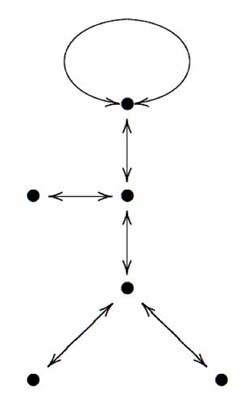If we consider the white vertices (which incidentally each lie on two edges as all points lying over 0 are of multiplicity two) as mid-points of longer edges connecting the
black vertices we obtain a drawing on the sphere which looks like \”Monsieur Mathieu\” but this time as a right handed bandit, and applying our mnemotechnic rule we obtain _another_ (non conjugated) embedding of $M_{12}$ in the full symmetric group on 12 vertices.

What is the connection with $SL_2(\mathbb{Z})$-representations? Well, the permutation generators s and t of $M_{12}$ (or $M_{24}$ for that matter) have orders two and three, whence there is a projection from the free group product $C_2 \star C_3$ (here $C_n$ is just the cyclic group of order n) onto $M_{12}$ (respectively $M_{24}$). Next
time we will say more about such free group products and show (among other things) that $PSL_2(\mathbb{Z}) \simeq C_2 \star C_3$ whence the connection with $SL_2(\mathbb{Z})$. In a following lecture we will extend the Monsieur Mathieu example to
arbitrary dessins d\’enfants which will allow us to assign to curves defined over $\overline{\mathbb{Q}}$ permutation representations of $SL_2(\mathbb{Z})$ and other _cartographic groups_ such as the congruence subgroups $\Gamma_0(2)$ and
$\Gamma(2)$.